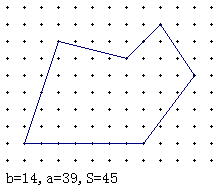## 皮克定理## 定理提出者

Georg Alexander Pick，1859年生于维也纳，1943年死于特莱西恩施塔特集中营

## 证明

### 多边形

PT的共同边上有c个格点。

• P的面积： iP + bP/2 - 1
• T的面积： iT + bT/2 - 1
• PT的面积：
(iT + iP + c - 2) + (bT- c + 2 + bP - c + 2 ) /2 - 1
= iPT + bPT/2 - 1

### 三角形

1. 所有平行于轴线的矩形；
2. 以上述矩形的两条邻边和对角线组成的直角三角形；
3. 所有三角形（因为它们都可内接于矩形内，将矩形分割成原三角形和至多3个第二点提到的直角三角形）。

#### 矩形

• AR = (m-1)(n-1)
• iR = (m-2)(n-2)
• bR = 2(m+n)-4
iR + bR/2 - 1
= (m-2)(n-2) + (m+n) - 2 - 1
= mn - (m + n) +1
= (m-1)(n-1)

#### 直角三角形

• b = m+n+c-3
• i = ((m-2)(n-2) - c + 2)/2
i + b/2 - 1
= ((m-2)(n-2) - c + 2)/2 + (m+n+c-3)/2 - 1
= (m-2)(n-2)/2 + (m+n - 3)/2
= (m-1)(n-1)/2

## 推广

• 取格点的组成图形的面积为一单位。在平行四边形格点，皮克定理依然成立。套用于任意三角形格点，皮克定理则是A = 2i + b - 2。
• 对于非简单的多边形P，皮克定理A = i + b/2 - χ(P)，其中χ(P)表示P的欧拉特征数。
• 高维推广：Ehrhart多项式；一维：植树问题。
• 皮克定理和欧拉公式（V-E+F=2）等价